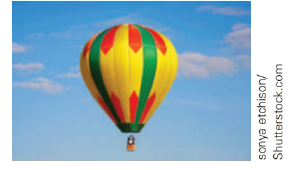Chapter 5.4, Problem 18EElementary Geometry For College St...

7th Edition
Alexander + 2 others
ISBN: 9781337614085

Solutions

Chapter
SectionElementary Geometry For College St...

7th Edition
Alexander + 2 others
ISBN: 9781337614085
Textbook Problem

A hot-air balloon is held in place by the ground crew at a point that is 21 ft from a point directly beneath the basket of the balloon. If the rope is of length 29 ft, how far above ground level is the basket?To determine

To Find:

Length of the basket above the ground level.

Explanation

Given A hot-air balloon is held in place by the ground crew at a point that is 21 ft from a point directly beneath the basket of the balloon. If the rope is of length 29 ft,

Let x be the length of the basket above the ground level. From the figure it is in form of right triangle in which one of the sides are 21 ft. and hypotenuse is 29 ft.

From the given data,

292=x2+212841=x

Still sussing out bartleby?

Check out a sample textbook solution.

See a sample solution

The Solution to Your Study Problems

Bartleby provides explanations to thousands of textbook problems written by our experts, many with advanced degrees!

Get Started

Find more solutions based on key concepts